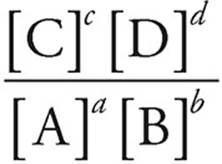﻿ ﻿THE REACTION QUOTIENT, Q - Equilibrium, Acids and Bases, Titrations, and Solubility - Content Review for the AP Chemistry Exam - Cracking the AP Chemistry Exam

## Cracking the AP Chemistry Exam

Part IV

Content Review for the AP Chemistry Exam

Chapter 8

Big Idea #6: Equilibrium, Acids and Bases, Titrations, and Solubility

THE REACTION QUOTIENT, Q

The reaction quotient is essentially the quantitative application of Le Châtelier’s Principle. It is determined using the law of mass action. However, you can use the concentration or pressure values at any point in the reaction to calculate Q. (Remember, you can only use concentrations or pressures of reactions at equilibrium to calculate K). The value for the reaction quotient can be compared to the value for the equilibrium constant to predict in which direction a reaction will shift from the given set of initial conditions.

The Reaction Quotient

For the reaction

aA+bBcC+dD

Q =[A], [B], [C], and [D] are initial molar concentrations or partial pressures.

· If Q is less than K, the reaction shifts right.

· If Q is greater than K, the reaction shifts left.

· If Q=K, the reaction is already at equilibrium.

Let’s take a look at an example. The following reaction takes place in a sealed flask:

2CH4(g)↔C2H2(g)+3H2(g)

(a) Determine the equilibrium constant if the following concentrations are found at equilibrium.

[CH4]=0.0032 M
[C2H2]=0.025 M
[H2]=0.040 M

To solve this, we first need to create the equilibrium constant expression:Plugging the numbers in, we get:

Kc =Kc =Kc = 0.16

(b) Upon testing this reaction at another point, the following concentrations are found:

[CH4]=0.0055 M

[C2H2]=0.026 M

[H2]=0.029 M

Use the reaction quotient to determine which way the reaction needs to shift to reach equilibrium.

Q uses the exact same ratios as the equilibrium constant expression, so:0.021 is less than the equilibrium constant value of 0.16, so the reaction must proceed to the right, creating more products and reducing the amount of reactant in order to come to equilibrium.

﻿